Courses

# RD Sharma Solutions -Ex-17.4, Symmetry, Class 6, Maths Class 6 Notes | EduRev

## RD Sharma Solutions for Class 6 Mathematics

Created by: Abhishek Kapoor

## Class 6 : RD Sharma Solutions -Ex-17.4, Symmetry, Class 6, Maths Class 6 Notes | EduRev

The document RD Sharma Solutions -Ex-17.4, Symmetry, Class 6, Maths Class 6 Notes | EduRev is a part of the Class 6 Course RD Sharma Solutions for Class 6 Mathematics.
All you need of Class 6 at this link: Class 6

Q. 1. The total number of lines of symmetry of a scalene triangle is

Sol: (d) none of these

This is because the line of symmetry of a scalene triangle is 0.

Q. 2. The total number of lines of symmetry of an isosceles triangle is

Sol: (a) 1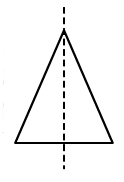Q. 3. An equilateral triangle is symmetrical about each of its

Sol: (d) all the above

In equilateral triangle altitudes, angle bisectors and medians are all the same.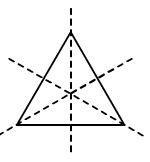Q. 4. The total number of lines of symmetry of a square is

Sol: (d) 4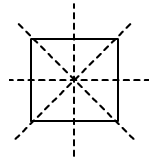Q. 5. A rhombus is symmetrical about

Sol: (a) Each of its diagonals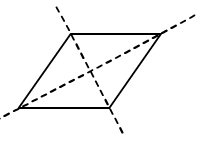Q. 6. The number of lines of symmetry of a rectangle is

Sol: (b) 2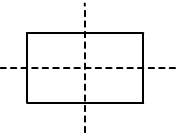Q. 7. The number of lines of symmetry of a kite is

Sol: (b) 1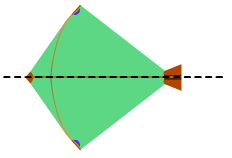Q. 8. The number of lines of symmetry of a circle is

Sol: (d) Unlimited

A circle has an infinite number of symmetry all along the diameters. It has an infinite number of diameters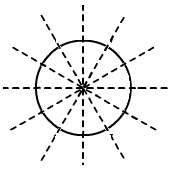Q. 9. The number of lines of symmetry of a regular hexagon is

Sol: (c) 6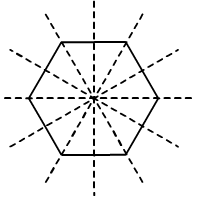Q. 10. The number of lines of symmetry of an n – sided regular polygon is

Sol: (a) n

The number of lines of symmetry of a regular polygon is equal to the sides of the polygon. If it has ‘n’ number of sides, then there are ‘n’ lines of symmetry

Q. 11. The number of lines of symmetry of the letter O of the English alphabet is

Sol: (c) 2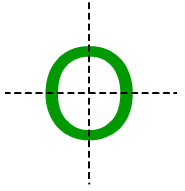Q. 12. The number of lines of symmetry of the letter Z of the English alphabet is

Sol: (a) 0

Z has no line of symmetry

103 docs

,

,

,

,

,

,

,

,

,

,

,

,

,

,

,

,

,

,

,

,

,

,

,

,

,

,

,

,

,

,

;